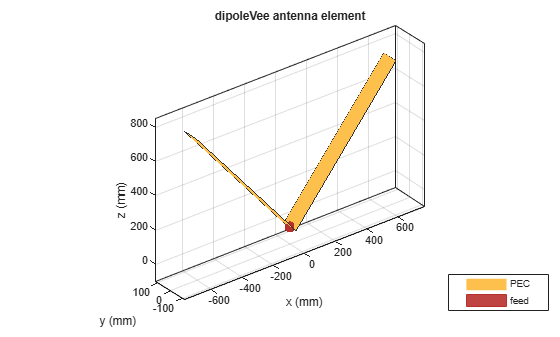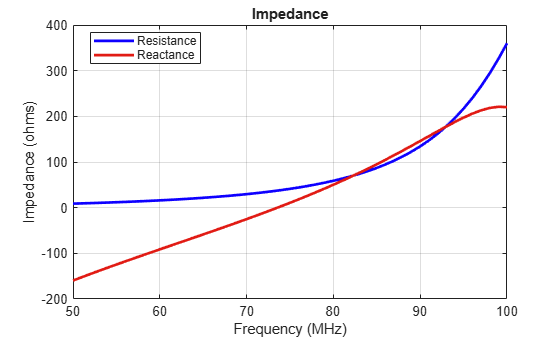Documentation

# dipoleVee

Create V-dipole antenna

## Description

The `dipoleVee` object is a planar V-dipole antenna in the X-Y plane.

The width of the dipole is related to the circular cross-section by the equation

`$w=2d=4r$`

, where:

• d is the diameter of equivalent cylindrical pole

• r is the radius of equivalent cylindrical pole

For a given cylinder radius, use the `cylinder2strip` utility function to calculate the equivalent width. The V-dipole antenna is bent around the feed point. The default V-dipole is center-fed and is in the X-Y plane. The feed point of the V-dipole antenna coincides with the origin.## Creation

### Syntax

``dv = dipoleVee``
``dv = dipoleVee(Name,Value)``

### Description

``` `dv = dipoleVee` creates a half-wavelength V-dipole antenna.```

example

``` `dv = dipoleVee(Name,Value)` creates a half-wavelength V-dipole antenna, with additional properties specified by one or more name-value pair arguments. `Name` is the property name and `Value` is the corresponding value. You can specify several name-value pair arguments in any order as `Name1`, `Value1`, `...`, `NameN`, `ValueN`. Properties not specified retain their default values.```

## Properties

expand all

Length of two arms, specified as a two-element vector in meters. By default, the arm lengths are chosen for an operating frequency of 75 MHz.

Example: `'ArmLength',[1,3]`

Data Types: `double`

V-dipole arm width, specified as a scalar in meters.

### Note

Dipole width should be less than `Total Arm Length`/5 and greater than `Total Arm Length`/1001. 

Example: `'Width',0.05`

Data Types: `double`

Angle made by two arms about X-Y plane, specified as a two-element vector in degrees.

Example: `'ArmElevation',[55 35]`

Data Types: `double`

Lumped elements added to the antenna feed, specified as a lumped element object handle. For more information, see `lumpedElement`.

Example: `'Load',lumpedelement`. `lumpedelement` is the object handle for the load created using `lumpedElement`.

Example: ```dv.Load = lumpedElement('Impedance',75)```

Tilt angle of the antenna, specified as a scalar or vector with each element unit in degrees. For more information, see Rotate Antennas and Arrays.

Example: `'Tilt',90`

Example: `'Tilt',[90 90]`,`'TiltAxis',[0 1 0;0 1 1]` tilts the antenna at 90 degree about two axes, defined by vectors.

Data Types: `double`

Tilt axis of the antenna, specified as:

• Three-element vectors of Cartesian coordinates in meters. In this case, each vector starts at the origin and lies along the specified points on the X-, Y-, and Z-axes.

• Two points in space, each specified as three-element vectors of Cartesian coordinates. In this case, the antenna rotates around the line joining the two points in space.

• A string input describing simple rotations around one of the principal axes, 'X', 'Y', or 'Z'.

Example: `'TiltAxis',[0 1 0]`

Example: `'TiltAxis',[0 0 0;0 1 0]`

Example: `ant.TiltAxis = 'Z'`

## Object Functions

 `show` Display antenna or array structure; Display shape as filled patch `info` Display information about antenna or array `axialRatio` Axial ratio of antenna `beamwidth` Beamwidth of antenna `charge` Charge distribution on metal or dielectric antenna or array surface `current` Current distribution on metal or dielectric antenna or array surface `design` Design prototype antenna or arrays for resonance at specified frequency `EHfields` Electric and magnetic fields of antennas; Embedded electric and magnetic fields of antenna element in arrays `impedance` Input impedance of antenna; scan impedance of array `mesh` Mesh properties of metal or dielectric antenna or array structure `meshconfig` Change mesh mode of antenna structure `pattern` Radiation pattern and phase of antenna or array; Embedded pattern of antenna element in array `patternAzimuth` Azimuth pattern of antenna or array `patternElevation` Elevation pattern of antenna or array `returnLoss` Return loss of antenna; scan return loss of array `sparameters` S-parameter object `vswr` Voltage standing wave ratio of antenna

## Examples

collapse all

Create and view a center-fed V-dipole that has 50 degree arm angles .

`dv = dipoleVee('ArmElevation',[50 50])`
```dv = dipoleVee with properties: ArmLength: [1 1] ArmElevation: [50 50] Width: 0.1000 Tilt: 0 TiltAxis: [1 0 0] Load: [1x1 lumpedElement] ```
`show(dv)`Calculate the impedance of a V-dipole antenna over the frequency range of 50MHz - 100MHz.

```dv = dipoleVee('ArmElevation',[50 50]); impedance(dv,linspace(50e6,100e6,51))```Balanis, C.A. Antenna Theory: Analysis and Design. 3rd Ed. New York: Wiley, 2005.

 Volakis, John. Antenna Engineering Handbook. 4th Ed. New York: McGraw-Hill, 2007.